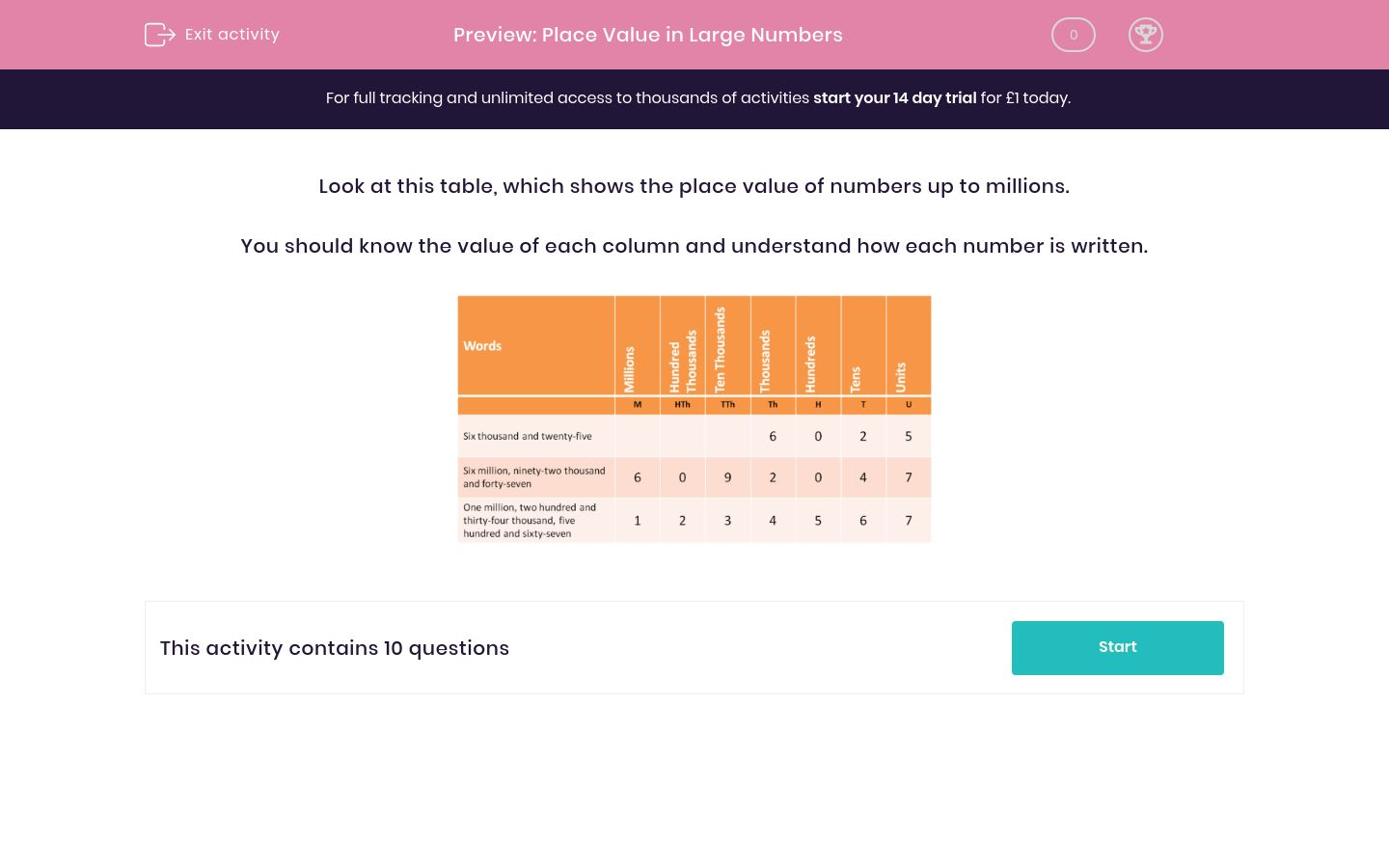# Place Value in Large Numbers

In this worksheet, students increase their fluency of reading numbers up to millions and state the column value of the given digits.Key stage:  KS 2

Curriculum topic:   Maths and Numerical Reasoning

Curriculum subtopic:   Place Value

Difficulty level:### QUESTION 1 of 10

Look at this table, which shows the place value of numbers up to millions.

You should know the value of each column and understand how each number is written.When you write the number 43124, select the column in which you would place the digit  3.

Hundreds

Thousands

Ten Thousands

Hundred Thousands

Millions

Tens

Units

When you write the number 38124, select the column in which you would place the digit  3.

Hundreds

Thousands

Ten Thousands

Hundred Thousands

Millions

Tens

Units

When you write the number 308124, select the column in which you would place the digit  3.

Hundreds

Thousands

Ten Thousands

Hundred Thousands

Millions

Tens

Units

When you write the number 5308124, select the column in which you would place the digit  3.

Hundreds

Thousands

Ten Thousands

Hundred Thousands

Millions

Tens

Units

When you write the number 5308124, select the column in which you would place the digit  2.

Hundreds

Thousands

Ten Thousands

Hundred Thousands

Millions

Tens

Units

When you write the number 5308124, select the column in which you would place the digit  5.

Hundreds

Thousands

Ten Thousands

Hundred Thousands

Millions

Tens

Units

When you write the number 5308124, select the column in which you would place the digit  8.

Hundreds

Thousands

Ten Thousands

Hundred Thousands

Millions

Tens

Units

When you write the number 507961, select the column in which you would place the digit  0.

Hundreds

Thousands

Ten Thousands

Hundred Thousands

Millions

Tens

Units

When you write the number 507961, select the column in which you would place the digit  9.

Hundreds

Thousands

Ten Thousands

Hundred Thousands

Millions

Tens

Units

When you write the number 5007001, select the column in which you would place the digit  5.

Hundreds

Thousands

Ten Thousands

Hundred Thousands

Millions

Tens

Units

• Question 1

When you write the number 43124, select the column in which you would place the digit  3.

Thousands
• Question 2

When you write the number 38124, select the column in which you would place the digit  3.

Ten Thousands
• Question 3

When you write the number 308124, select the column in which you would place the digit  3.

Hundred Thousands
• Question 4

When you write the number 5308124, select the column in which you would place the digit  3.

Hundred Thousands
• Question 5

When you write the number 5308124, select the column in which you would place the digit  2.

Tens
• Question 6

When you write the number 5308124, select the column in which you would place the digit  5.

Millions
• Question 7

When you write the number 5308124, select the column in which you would place the digit  8.

Thousands
• Question 8

When you write the number 507961, select the column in which you would place the digit  0.

Ten Thousands
• Question 9

When you write the number 507961, select the column in which you would place the digit  9.

Hundreds
• Question 10

When you write the number 5007001, select the column in which you would place the digit  5.

Millions
---- OR ----

Sign up for a £1 trial so you can track and measure your child's progress on this activity.

### What is EdPlace?

We're your National Curriculum aligned online education content provider helping each child succeed in English, maths and science from year 1 to GCSE. With an EdPlace account you’ll be able to track and measure progress, helping each child achieve their best. We build confidence and attainment by personalising each child’s learning at a level that suits them.

Get started